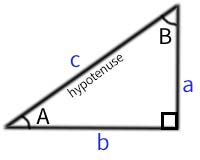## Conveyor Calculation Tools

### Right Angle Triangle Calculator

When using a grip faced belt the maximum incline angle is 23°

Show values to . . . significant figures.

 edge a = units edge b = units edge c = units angle A = degrees angle B = degrees Total area = square units